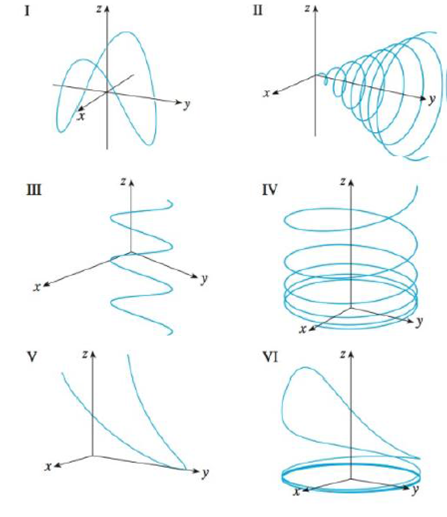Chapter 13.1, Problem 25E

Chapter
Section
Textbook Problem

# Match the parametric equations with the graphs (labeled I-VI). Give reasons for your choices.25. x = cos 8t, y = sin 8t, z = e0.8t, t ≥ 0

To determine

To match: The parametric equations with graphs.

Explanation

Given data:

The parametric equations x=cos8t , y=sin8t , and z=e0.8t .

Find the value of x2+y2 .

x2+y2=(cos8t)2+(sin8t)2=cos28t+sin28t{cos2x+sin2x=1}=1

The equation x2+y2=1 indicates that curve lies on circular cylinder with z-plane

### Still sussing out bartleby?

Check out a sample textbook solution.

See a sample solution

#### The Solution to Your Study Problems

Bartleby provides explanations to thousands of textbook problems written by our experts, many with advanced degrees!

Get Started